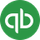cancel
Showing results for
Did you mean:Intuit

# Set up asset accounts to track depreciation in QuickBooks Desktop for Mac

Learn how to set up fixed assets to track depreciation in QuickBooks Desktop for Mac.

There are several ways to track fixed assets and depreciation. This is one suggestion.

## What are assets?

Company assets are the things that your company owns.

Here's the difference between two types of assets:

• Current assets: These are the assets you’re likely to convert to cash within one year. They include the cash you have on hand, the money in your checking and savings accounts, and the money your customers owe your business.
• Fixed assets: These are the assets you don’t expect to convert to cash during one year of normal operations. A fixed asset is usually something that is necessary for the operation of your business—like a truck, cash register, or computer.

## Depreciation and accumulated depreciation

Depreciation is the decline in the value of a physical asset while accumulated depreciation is the cumulative depreciation of an asset up to a single point in its life.

## Set up an asset account to track depreciation

1. Create a fixed asset account for each asset (or group of assets) you want to depreciate. The account can represent either a single asset or a group of related assets.
1. Make the account type “Fixed Asset.”
2. Give each account a name that indicates which asset(s) it is tracking, for example, truck, office furniture, computer.
3. Leave the opening balance at 0.00.
2. Add two subaccounts to each asset account you created. One subaccount tracks the cost of the asset, the other tracks accumulated depreciation.
1. Each main account must have two subaccounts.
2. Name the first subaccount something like “Cost” and the second subaccount something like “Accumulated Depreciation.” In your chart of accounts, these names will help you distinguish the two subaccounts.
3. For the Cost subaccount, enter the original cost of the asset in the Opening Balance field. Enter the date you purchased the asset in the As of field.
4. For the Accumulated Depreciation subaccount, type 0.00 as the opening balance if you acquired the asset after your QuickBooks start date. If you acquired the asset before the start date, enter instead the accumulated depreciation of the asset AS OF the start date. Enter this amount as a negative number.
3. Create an expense account to track depreciation expense. Give the account a name like “Depreciation Expense.”

## Enter a depreciation

Important: Determining the amount of depreciation to deduct can be a complex process, and the IRS rules on the subject change often. Ask an accountant for help in figuring actual depreciation amounts.

1. Go to Lists, then select Chart of Accounts.
2. Select the subaccount that tracks accumulated depreciation for the asset you're depreciating.
3. Select Use Register from the Action pop-up menu.
4. Enter the transaction in the bottom of the register:
• Enter the depreciation amount as a decrease in the register.
• In the Account field, enter the expense account you set up to track depreciation.

Note: In the main fixed asset account, QuickBooks subtracts the depreciation amount from the current value of the asset. In the account that tracks depreciation, QuickBooks enters the depreciation amount as an increase to your company's depreciation expense.